CONTRAST Statement

CONTRAST ’label’ contrast-specification <, contrast-specification> <, …> </ options> ;

The CONTRAST statement provides a mechanism for obtaining custom hypothesis tests. It is patterned after the CONTRAST statement in PROC MIXED and enables you to select an appropriate inference space (McLean, Sanders, and Stroup, 1991).

You can test the hypothesis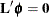, where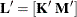and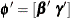, in several inference spaces. The inference space corresponds to the choice of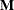. When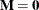, your inferences apply to the entire population from which the random effects are sampled; this is known as the broad inference space. When all elements ofare nonzero, your inferences apply only to the observed levels of the random effects. This is known as the narrow inference space, and you can also choose it by specifying all of the random effects as fixed. The GLM procedure uses the narrow inference space. Finally, by zeroing portions ofcorresponding to selected main effects and interactions, you can choose intermediate inference spaces. The broad inference space is usually the most appropriate, and it is used when you do not specify any random effects in the CONTRAST statement.

In the CONTRAST statement,

label

identifies the contrast in the table. A label is required for every contrast specified. Labels can be up to 20 characters and must be enclosed in single quotes.

contrast-specification

identifies the fixed effects and random effects and their coefficients from which thematrix is formed. The syntax representation of a contrast-specification is <fixed-effect values …> <| random-effect values …>

fixed-effect

identifies an effect that appears in the MODEL statement. The keyword INTERCEPT can be used as an effect when an intercept is fitted in the model. You do not need to include all effects that are in the MODEL statement.

random-effect

identifies an effect that appears in the RANDOM statement. The first random effect must follow a vertical bar (|); however, random effects do not have to be specified.

values

are constants that are elements of thematrix associated with the fixed and random effects.

The rows of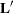are specified in order and are separated by commas. The rows of the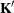component ofare specified on the left side of the vertical bars (|). These rows test the fixed effects and are, therefore, checked for estimability. The rows of the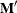component ofare specified on the right side of the vertical bars. They test the random effects, and no estimability checking is necessary.

If PROC HPMIXED finds the fixed-effects portion of the specified contrast to be nonestimable (see the SINGULAR= option), then it displays missing values for the test statistics and a note in the log.

If the elements ofare not specified for an effect that contains a specified effect, then the elements of the specified effect are automatically filled in over the levels of the higher-order effect. This feature is designed to preserve estimability for cases where there are complex higher-order effects. The coefficients for the higher-order effect are determined by equitably distributing the coefficients of the lower-level effect as in the construction of least squares means. In addition, if the intercept is specified, it is distributed over all classification effects that are not contained by any other specified effect. If an effect is not specified and does not contain any specified effects, then all of its coefficients inare set to 0. You can override this behavior by specifying coefficients for the higher-order effect.

If too many values are specified for an effect, the extra ones are ignored; if too few are specified, the remaining ones are set to 0. If no random effects are specified, the vertical bar can be omitted; otherwise, it must be present. If a SUBJECT effect is used in the RANDOM statement, then the coefficients specified for the effects in the RANDOM statement are equitably distributed across the levels of the SUBJECT effect. You can use the E option to see exactly whatmatrix is used.

The SUBJECT and GROUP options in the CONTRAST statement are useful for the case where a SUBJECT= or GROUP= variable appears in the RANDOM statement, and you want to contrast different subjects or groups. By default, CONTRAST statement coefficients about random effects are distributed equally across subjects and groups.

PROC HPMIXED handles missing level combinations of CLASS variables similarly to the way PROC GLM does. Both procedures delete fixed-effects parameters corresponding to missing levels in order to preserve estimability. However, PROC HPMIXED does not delete missing level combinations for random-effects parameters because linear combinations of the random-effects parameters are always estimable. These conventions can affect the way you specify your CONTRAST coefficients.

The CONTRAST statement computes the statistic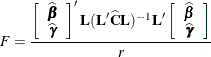where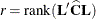and approximates its distribution with an F distribution. In this expression,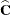is an estimate of the generalized inverse of the coefficient matrix in the mixed model equations.

The numerator degree of freedom in the F approximation is, and the denominator degree of freedom is taken from the Type III Tests of Fixed Effects table and corresponds to the final effect you list in the CONTRAST statement. You can change the denominator degrees of freedom by using the DF= option.

You can specify the following options in the CONTRAST statement after a slash (/).

CHISQ

requests thattests be performed in addition to any F tests. Astatistic equals its corresponding F statistic times the associate numerator degree of freedom, and this same degree of freedom is used to compute the p-value for thetest. This p-value will always be less than that for the F test, as it effectively corresponds to an F test with infinite denominator degrees of freedom.

DF=number

specifies the denominator degrees of freedom for the F test. The default is the denominator degrees of freedom taken from the Type III Tests of Fixed Effects table and corresponds to the final effect you list in the CONTRAST statement.

E

requests that thematrix coefficients for the contrast be displayed. The name of this L Matrix Coefficients table is Coef.

GROUP coeffs

sets up random-effect contrasts between different groups when a GROUP= variable appears in the RANDOM statement. By default, CONTRAST statement coefficients about random effects are distributed equally across groups. If you enter a multi-row contrast, you can also enter multiple rows for the GROUP coefficients. If the number of GROUP coefficients is less than the number of contrasts in the CONTRAST statement, the HPMIXED procedure cycles through the GROUP coefficients. For example, the following two statements are equivalent:

contrast 'Trt @ x=0.4 and 0.5' trt 1 -1  0 | x 0.4,
trt 1  0 -1 | x 0.4,
trt 1 -1  0 | x 0.5,
trt 1  0 -1 | x 0.5 /
group 1 -1, 1 0 -1, 1 -1, 1 0 -1;

contrast 'Trt @ x=0.4 and 0.5' trt 1 -1  0 | x 0.4,
trt 1  0 -1 | x 0.4,
trt 1 -1  0 | x 0.5,
trt 1  0 -1 | x 0.5 /
group 1 -1, 1 0 -1;
SINGULAR=number

tunes the estimability checking. Ifis a vector, define ABS() to be the largest absolute value of the element ofwith the largest absolute value. If ABS(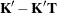) is greater than c*number for any row ofin the contrast, then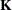is declared nonestimable. Here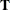is the Hermite form matrix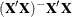, and c is ABS() except when it equals 0, and then c is 1. The value for number must be between 0 and 1; the default is 1E–4.

SUBJECT coeffs

sets up random-effect contrasts between different subjects when a SUBJECT= variable appears in the RANDOM statement. By default, CONTRAST statement coefficients about random effects are distributed equally across subjects. Listing subject coefficients for multiple row CONTRASTS follows the same rules as for GROUP coefficients.